##### Hot Keywords

TopJ Mater Inf 2022;2:11. 10.20517/jmi.2022.15 © The Author(s) 2022.
Open Access Research Article

# A thermodynamic database of the Ni-Mo-Re system

School of Materials Science and Engineering, Shanghai University, Shanghai 200444, China.

Materials Genome Institute, Shanghai University, Shanghai 200444, China.

State Key Laboratory of Powder Metallurgy, Central South University, Changsha 410083, Hunan, China.

*Correspondence to: Prof. Xiao-Gang Lu, School of Materials Science and Engineering, Shanghai University, No. 333 Nanchen Road, Shanghai 200444, China. E-mail: xglu@t.shu.edu.cn

Views:293 | Downloads:251 | Cited:0 | Comments:0 | :1
Academic Editors: Xingjun Liu, Lijun Zhang | Copy Editor: Tiantian Shi | Production Editor: Tiantian Shi

## Abstract

Thermodynamic databases are essential prerequisites for developing advanced materials, such as Ni-based superalloys. The present work collects a large amount of experimental and first-principles calculation data concerning the thermodynamics and phase diagrams of the Ni-Mo-Re system, based on which the thermodynamic properties of the ternary and its binary sub-systems Ni-Mo and Mo-Re are assessed by means of the CALculation of PHAse Diagrams (CALPHAD) approach. The thermodynamic database containing all model parameters is established and most experimental data are reproduced satisfactorily. The present work demonstrates the use of the CALPHAD method as a practical appliance in the toolbox of materials informatics to analyze and discriminate various types of data by thermodynamic modeling and then produce more useful data in wider ranges of compositions and temperatures by computational predictions.

## INTRODUCTION

Ni-based superalloys play a significant role in high-temperature applications for modern industry. Refractory alloying elements, such as Mo and Re, are often added to enhance the high-temperature performance of Ni-based superalloys further. However, some harmful brittle topologically close-packed (TCP) phases may form with increasing contents of alloying elements. In order to understand the phase stabilities of TCP phases, as well as their equilibria with other phases, it is necessary to develop a reliable thermodynamic database to predict phase equilibria and transformations in Ni-based superalloys, starting from ternaries, such as Ni-Mo-Re.

The phase diagram and thermodynamics of the Ni-Mo-Re ternary system were studied by Yaqoob and Joubert[2,3] and the enthalpies of formation of compounds were calculated by Crivello by means of first-principles calculations. A new phase with a modulated $$\sigma$$ phase structure was reported and named as the $$\sigma$$' phase by Yaqoob et al.. The average structure of this phase is the $$\sigma$$ phase, existing in the binary Mo-Re system and extending to the ternary Ni-Mo-Re system. Further structural characterization of this compound could not be completed because of the poor quality of the sample. Yaqoob treated the phase as a line compound, (Mo, Re)$$_ \rm{2}$$(Ni)$$_1$$, without considering its structural relationship with the $$\sigma$$ phase and ignoring the experimentally determined solubility range of Ni from $$\sim$$26 to $$\sim$$33 at.%. There exist other limitations in the assessment by Yaqoob regarding the subsystems, the extension of the binary $$\delta$$ phase in the ternary system and the liquidus projection.

The present work aims to obtain a set of self-consistent thermodynamic parameters of the Ni-Mo-Re system with more inputs from first-principles calculations. The binary systems Ni-Mo and Mo-Re are revised to obtain a better agreement with the experimental data. By combining the thermodynamic description of the Ni-Re system reported by Yaqoob and Joubert with the updated Ni-Mo and Mo-Re systems, the reassessment of the Ni-Mo-Re ternary system is carried out.

## LITERATURE REVIEW

All available experimental studies[3,7-46] are summarized in Table 1.

Table 1

Performance accuracy (%) with patch images

 Type of data Reference Method Quoted mode$$^ \rm{a}$$ TA: Thermal analysis; OM: optical metallography; XRD: X-ray diffraction; CA: chemical analysis; DTA: differential thermal analysis; DC: diffusion couple; EPMA: electron probe microanalysis; EPS: electrolytic phase separation; EA: equilibrated alloys; DM: diffusion multiple; AC: adiabatic calorimetry; EMF: electromotive force; FP: first-principles calculations; VSM: vibrating sample magnetometer; SQUID: superconducting quantum interference device. aIndicates whether the data are used or not in the assessment: $$\bullet$$ used; $$\circ$$ : used partially; ▎: not used. Ni-Mo binary system Phase equilibria 70-85 at.% Ni Grube et al. ▎ 60-76 at.% Ni Bloom et al. $$\bullet$$ fcc Guthrie et al. $$\circ$$ Overall Casselton et al. TA, OM, XRD $$\circ$$ bcc liquidus Wicker et al. CA, DTA $$\bullet$$ Below 1600 K Heijwegen et al. DC, EPMA $$\bullet$$ fcc Gust et al. (Not available) $$\bullet$$ bcc solidus Kang et al. EPS, EPMA $$\bullet$$ fcc + Ni4Mo Kobayashi et al. XRD $$\bullet$$ bcc solidus, fcc + $$\delta$$ Yaqoob et al. EA, EPMA $$\bullet$$ 1173K and 1073K Zhu et al. DM, EPMA $$\bullet$$ 1373K and 1123K Shi et al. DC, EA, SEM, XRD $$\bullet$$ Thermodynamic properties Enthalpy of mixing of liquid Chistyakov et al. Calorimetry $$\bullet$$ Sudavtsova Calorimetry ▎ Enthalpy of formation for$$\delta$$, heat capacity for $$\delta$$ Spencer et al. AC $$\bullet$$ Enthalpies of formation for $$\delta$$ Kubaschewski et al.  AC $$\bullet$$ Heat capacity of Ni$$_4$$Mo Norem AC $$\bullet$$ Brook et al. AC ▎ Basak AC $$\bullet$$ Activity Katayama et al. EMF ▎ Meshkov et al. EMF $$\bullet$$ Tsai et al. EMF $$\bullet$$ Pejryd[28,29] EMF ▎ Koyama et al. EMF $$\bullet$$ Sudavtsova EMF ▎ Curie temperature Khan et al. VSM, SQUID $$\bullet$$ Magnetic moment Ghosh et al. VSM, SQUID $$\bullet$$ Mo-Re system Phase equilibria Overall Dickinson et al. OM, XRD $$\bullet$$ Knapton et al. OM, XRD $$\bullet$$ Savitskii et al.[38,39] OM, XRD $$\bullet$$ Thermodynamic properties 1473K and 1873K, enthalpy of formation Farzadfar et al. OM, XRD, DRC $$\bullet$$ Ni-Mo-Re system Phase equilibria 1425K Kodentsov et al. DC ▎ 1425K Borisov et al.  Slyusarenko et al. DC & EA $$\bullet$$ 1473K Feng et al. DM ▎ 1473K and 1873K, liquidus projection Yaqoob et al. EA, EPMA, XRD $$\bullet$$ Thermodynamic properties Site occupancy of $$\sigma$$ Yaqoob et al. XRD $$\bullet$$ Heat capacity of NMR-75 Chekhovskoi et al. AC ▎

### Ni-Mo

The Ni-Mo system has been extensively investigated by experiments[3,7-33] and thermodynamic modeling[32,33,47-49]. Due to the lack of experimental and first-principles calculated thermodynamic data, earlier thermodynamic assessments[47,48] are less reliable considering the formation enthalpies of some intermetallic compounds. Morishita et al. treated the $$\delta$$ phase as a stoichiometric compound, which is inconsistent with the homogeneity domain of 4 at.%. With the aid of first-principles calculations (some of the data were calculated by Wang et al.), Zhou et al. obtained a set of thermodynamic parameters of the Ni-Mo system by the CALPHAD method[31,32]. However, overfitting with too many parameters to the phase diagram at low temperatures led to a much larger heat capacity $$(C_P)$$ deviation from the experimental data[20,22]. Later, Yaqoob et al. reviewed the experimental information critically and reassessed the Ni-Mo system. The number of parameters was reduced significantly compared with those of Zhou et al.. However, the modeling of Yaqoob et al. can be further improved considering the following issues: (1) the enthalpy of mixing of the liquid phase is not in agreement with the experimental data[18,19]; (2) the enthalpy of formation of the $$\delta$$ phase at high temperature is lower than the experimental data[20,21]; (3) for the fcc phase, the SQS calculated enthalpy, the experimental Gibbs energy of mixing and the $$C_P$$ at high temperature cannot be reproduced and (4) the magnetic properties of the fcc phase were ignored.

### Mo-Re

Thermodynamic assessments of the Mo-Re system have been published by many authors[50-53]. Mathieu et al. reviewed all the previous work and performed reassessments aided by first-principles calculations. A full five-sublattice (5SL) model and various simpliﬁed sublattice models of the $$\sigma$$ phase were considered. Compared with the previous assessments, the thermodynamic properties calculated by Mathieu et al. show better agreement with the first-principles calculation data. However, the homogeneity range of the $$\sigma$$ phase at 1473 K calculated by Mathieu et al. (as well as other previous assessments) was very narrow. This is in contrast to the experimental data for not only the binary but also the ternary system. One may argue the accuracy of the experiments but it deserves a detailed study as discussed below. Moreover, when extrapolating the Mo-Re system with the parameters of Mathieu et al. to the ternary system, the Mo content in the bcc phase of the three-phase equilibria (i.e., bcc + $$\delta$$ + Mo$$_3$$CoSi and bcc + Mo$$_3$$CoSi + $$\sigma$$) was significantly higher than the experimental data, which is caused by a large value of $$^1L_{Mo, Re}^{bcc}$$ used to fit the equilibria between the bcc and $$\sigma$$ phases.

### Ni-Re

The experimental information of the Ni-Re binary system before 1985 has been reviewed critically by Nash et al. and an assessed Ni-Re phase diagram was reported. Later, Yaqoob and Joubert reinvestigated experimentally and reassessed this binary system, and agreed well with most experimental data. Furthermore, the invariant reaction temperature and corresponding compositions determined by Yaqoob and Joubert were reconfirmed by Boettinger et al.. Thus, the thermodynamic parameters from Yaqoob and Joubert were directly employed in the present work.

### Ni-Mo-Re

Several experimental investigations have been carried out on the phase equilibria of the Ni-Mo-Re system[3,42,43,44]. Their measured phase diagrams are quite different in terms of the number and homogeneity ranges of the ternary intermetallic compounds.

The experimental data mainly considered in the present work are the phase equilibria of the Ni-Mo-Re system at 1473 and 1873 K determined by Yaqoob and Joubert utilizing EPMA and XRD. The equilibrium annealing treatments for the alloys were at 1873 K for 9 h and at 1473 K for 70 d. The crystal structure identification of the ternary intermetallic compounds was carried out and two unknown ternary compounds were observed. One phase has a body-centered tetragonal crystal structure with a structure prototype Mo$$_3$$CoSi (named as bct in the present work) and the other has a modulated $$\sigma$$ structure. The isothermal section measured by Yaqoob and Joubert showed a large extension of the $$\sigma$$ and $$\delta$$ phases and a limited extension of the $$x$$ phase. The long annealing time and phase identification technique ensured the reliability of their work so that their result was used in the present work.

The phase equilibria at 1425 K were determined by Borisov et al. and Slyusarenko et al. using equilibrated alloys. The annealing time for the samples was 33.3 d[42,43]. The obtained isothermal section shows good compatibility with the work of Yaqoob and Joubert, except that only one ternary intermetallic compound was found. Borisov et al. and Slyusarenko et al. probably misinterpreted two phases as one single phase without performing phase identification[42,43]. Generally, these experimental data are considered reliable data.

The isothermal sections proposed by Kodentsov et al. and Feng et al. show poor agreement with that from Yaqoob and Joubert and are therefore not utilized in the present assessment[41,44].

The site occupancies of the Ni-Mo-Re $$\sigma$$ phase were determined by Yaqoob et al. as a function of composition in the ternary homogeneity domain, which were considered in the present work.

Chekhovskoi et al. measured the CP of the NMR-75 alloy (75 wt.% Ni, 15 wt.% Mo and 10 wt.% Re) in a temperature range of 300-1300 K. However, the results were not used in the present work since it was difficult to reproduce if other data were to be considered simultaneously.

### FIRST-PRINCIPLES CALCULATIONS

First-principles calculations were performed using the Vienna ab initio simulation package (VASP) with plane-wave basis sets based on density functional theory (DFT). Projector augmented wave pseudopotentials were adopted to represent the valence electrons. GGA-PW91 was adopted to describe the exchange and correlation effects. A large plane-wave cutoff energy of 400 eV was set for the plane-wave basis. The Monkhorst-Pack scheme was used to construct the k-point meshes for Brillouin zone sampling. The spin-polarized calculations were performed with ferromagnetism set. The convergence criteria for electronic self-consistency and ionic minimization were 10$$^{-5}$$ and 10$$^{-4}$$ eV/unit cell, respectively. After relaxation, a final static calculation was conducted by employing the tetrahedron method with Blöchl corrections.

For the bcc, fcc and hcp solid solutions, the SQS method[62,63] was adopted to generate disordered structures for the VASP calculations. Supercells containing 16 atoms were constructed considering the configurations A12B4 and A15B1, where "A" denotes Ni for fcc, Mo for bcc and Re for hcp. Note that possible unstable structures, such as fcc Mo15Ni1, were not calculated since the full relaxation may not maintain the original structures. The total number of k-points multiplied by the total number of atoms per unit cell was at least 7200 for fcc, 6336 for bcc and 8000 for hcp. Using VASP, the SQS supercells were first relaxed with respect to cell volume only, then to volume and cell shape, and finally simultaneously to volume, cell shape and atomic position (i.e., full relaxation) in the present work. On this basis, a fast convergence speed can be achieved. To check the symmetry preservation, the radial distribution functions were analyzed.

In addition, VASP calculations were also performed to obtain the formation energies of all the end-members of the $$\sigma$$ and $$x$$ phases. The k-point meshes were 12 × 12 × 12 and 8 × 8 × 15, respectively. To facilitate the calculations, the ZenGen script-tool was adopted to conveniently generate input files for the VASP calculations.

## THERMODYNAMIC MODELS

In the Ni-Mo-Re system, the stable phases included in this assessment are liquid, fcc, bcc, hcp, $$\delta$$, Ni$$_3$$Mo, Ni$$_4$$Mo, $$\sigma$$, $$x$$ and the bct phase with a Mo$$_3$$CoSi structural prototype. Their crystal structure information and the thermodynamic models are summarized in Table 2. The thermodynamic descriptions of the pure elements Ni, Mo and Re were taken from the Scientific Group Thermodata Europe (SGTE) unary database (Version 5.0). All the phases were described by the sublattice model within CEF. Detailed mathematical expressions are available in our previous work.

Table 2

Crystallographic and model information of stable phases in Ni-Mo-Re system

 Phase Pearson symbol Prototype Model Liquid - - (Ni, Mo, Re)$$_1$$ fcc_A1 cF4 Cu (Ni, Mo, Re)$$_1$$(VA)$$_1$$ bcc_A2 cI2 W (Ni, Mo, Re)$$_1$$(VA)$$_3$$ hcp_A3 hP2 Mg (Ni, Mo, Re)$$_1$$(VA)$$_{0.5}$$ $$\delta$$ oP56 NiMo (Ni)$$_{24}$$(Ni, Mo, Re)$$_{20}$$(Mo)$$_{12}$$ Ni$$_3$$Mo oP8 Cu$$_3$$Ti (Ni, Mo)$$_3$$(Ni, Mo)$$_1$$ Ni$$_4$$Mo tI10 Ni$$_4$$Mo (Ni)$$_4$$(Mo)$$_1$$ $$\sigma$$ tP30 CrFe Enthalpy of formation for$$\delta$$, heat capacity for $$\delta$$ $$x$$ cI58 $$\alpha$$-Mn 1473K and 1873K, enthalpy of formation bct tI56 Mo$$_3$$CoSi (Mo, Re)$$_9$$(Ni, Re)$$_4$$(Ni)$$_1$$

### Solution phases

The solution phases (including liquid, fcc, bcc and hcp) were treated as substitutional solutions and described by a substitutional solution model with the Redlich-Kister polynomials.

Normally, for the interaction parameter, a linear temperature dependence is sufficient, i.e., $$^nL^{\varphi}_{i, j} + ^na_{i, j} + ^nb_{i, j}T$$. In the present work, the measured excess heat capacity for the fcc phase of the Ni-Mo system is available, so a $$Tln(T)$$ term was introduced to produce a constant excess heat capacity.

#### Intermetallic phases

In the present work, three intermetallic phases, i.e., $$\delta$$, Ni$$_3$$Mo and Ni$$_4$$Mo, were considered for the Ni-Mo system. The solubility of Re was ignored in both Ni$$_3$$Mo and Ni$$_4$$Mo due to the lack of experimental data.

The molar Gibbs energy of each intermetallic phase $$\varphi$$ is described as:

$$G_{m}^{\varphi}=\sum\limits_{I} y_{i}^{s}\, ^oG_{I}^{\varphi}+R T\left(\sum\limits_{s} a^{s} \sum\limits_{i} y_{i}^{s} \ln y_{i}^{s}\right)+{ }^{e x} G_{m}^{\varphi} ;$$

where $$y_{i}^{s}$$ is the site fraction of component i in the sublattice s, $$^oG_{I}^{\varphi}$$ is the Gibbs free energy of the end member I, R is the gas constant, $$a^{s}$$ as is the number of sites of sublattice s and $${ }^{e x} G_{m}^{\varphi}$$ corresponds to the molar excess Gibbs energy, expressed in the Redlich-Kister polynomial.

Although the Ni$$_3$$Mo compound with the D0$$_a$$ structure is stoichiometric in the Ni-Mo system, the phase with the same structure is non-stoichiometric in some ternary systems, such as Ni-Mo-Ta, modeled as (Ni, Mo, Ta)$$_3$$(Ni, Mo, Ta)$$_1$$ by Cui et al.. To extend to high-order systems for a multi-component Ni-based database, the two-sublattice model of (Ni, Mo)$$_3$$(Ni, Mo)$$_1$$ was also adopted in the present work.

For non-stoichiometric phase Ni$$_3$$Mo, the Kopp-Neumann rule was applied to estimate the $$C_P$$ of end-members based on the composition average of the $$C_P$$ of the pure elements in their stable element reference (SER) at 298 K, i.e., fcc Ni, bcc Mo and hcp Re. Its Gibbs energy per mole of the formula of the end-members can be expressed as:

$${ }^{o} G_{i : j}^{N i, M o}=3^{o} G_{i}^{S E R}+{ }^{o} G_{j}^{S E R}+a+b T$$

Following the previous assessment for the Ni-Mo binary, the $$\delta$$ phase was modeled as (Ni)$$_{24}$$(Ni, Mo)$$_{20}$$(Mo)$$_{12}$$, a simplified model of (Ni)$$_{24}$$(Ni, Mo)$$_{20}$$(Mo)$$_8$$(Mo)$$_4$$. The coordination numbers (CNs) of the three sublattices are 12, 14 and 15/16, respectively. Since the atomic size of Re is medium among the three elements, Yaqoob assumed that Re only occupies the second sublattice (i.e., CN = 14), which was adopted in the present work. Therefore, the model for the $$\delta$$ phase was extended to (Ni)$$_{24}$$(Ni, Mo, Re)$$_{20}$$(Mo)$$_{12}$$.

The end-members of the $$\delta$$ phase can be described by Eq. (3), in which the parameter a was fixed to the enthalpy data from Zhou et al. (except that of the end-member Ni: Re: Mo, which was quoted from Yaqoob) and b is the entropy term to be evaluated based on phase equilibria. As the enthalpy data measured at high temperature are available, a TlnT term may be needed as follows:

$${ }^{o} G_{i: j: k}^{\delta}=24^{o} G_{i}^{S E R}+20^{o} G_{j}^{S E R}+12^{o} G_{k}^{S E R}+a+b T+c T \ln T$$

Because of the limited solubility range reported by Heijwegen and Rieck, Kobayashi et al. and Zhu et al., the Ni$$_4$$Mo phase was treated as a stoichiometric compound[15,16]. Since $$C_P$$ for the Ni$$_4$$Mo phase is known, the Gibbs energy per mole of formula Ni$$_4$$Mo is parameterized to fit the $$C_P$$ data with a power series in temperature as follows:

$$G_{m}^{Ni_4Mo}=a+b T+c T \ln T + dT^2$$

where the parameters c and d were obtained from the $$C_P$$ data and the parameters a and b were evaluated based on phase equilibria and enthalpy data.

There exist two intermetallic phases (i.e., $$\sigma$$ and $$x$$) in the binary Mo-Re system. The $$\sigma$$ phase is the most important phase in the Ni-Mo-Re system. Ideally, the $$\sigma$$ phase should be modeled as a 5SL model. In contrast, the simplified three-sublattice model (3SL), i.e., (Ni, Mo, Re)$$_{10}$$(Ni, Mo, Re)$$_{4}$$(Ni, Mo, Re)$$_{16}$$ is also widely used to reduce the end-members. Figure 1 shows the calculated Gibbs energy of the $$\sigma$$ phase at 298 K along 0.5Mo0.5Re-Ni and binary Mo-Re using the 5SL and 3SL models, respectively, with the formation enthalpies of all the end-members in Eq. (1) determined by VASP data calculated in the present work. Obviously, the 5SL model manifests two composition sets of the $$\sigma$$ phase in the ternary system and theoretically predicts the composition sets, which is consistent with the experimental finding of Yaqoob et al..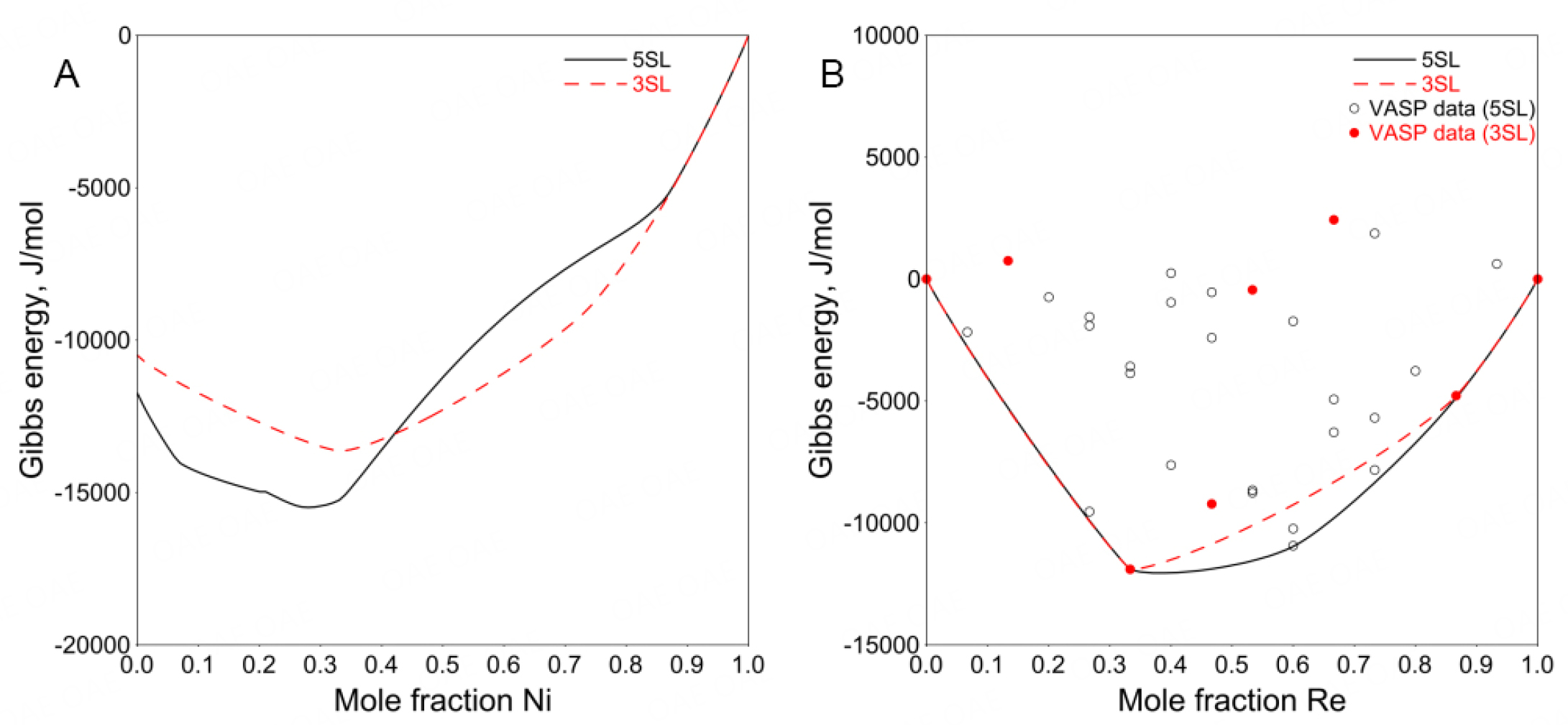Figure 1. Calculated Gibbs energy of $$\sigma$$ phase at 298 K along (A) 0.5Mo0.5Re-Ni and (B) Mo-Re using the 5SL and 3SL models compared with the VASP results. Sigma Ni, sigma Mo and sigma Re are used as reference states.

The simplified 3SL fails to capture this structural relationship using the VASP data (mainly representing nearest-neighbor interactions) only. One can use positive interaction parameters within a sublattice to produce the composition sets. However, since there are only 23 binary end-members in the 3SL model, no suitable interaction parameters can be used to adjust the composition sets according to the experimentally determined composition range. For a detailed explanation, see Appendix I.

Therefore, the 5SL model combined with the VASP data was adopted in the present work to ensure reasonable modeling of the $$\sigma$$ phase and its modulated counterpart.

The Gibbs energy of an end-member of $$\sigma$$ is expressed as follows:

$${ }^{o} G_{A B C D E}^{\sigma}=\sum\limits_{s} a^{s}{ } ^{o} G_{i}^{S E R}+E_{A B C D E}^{\sigma}-\sum\limits_{s} a^{s} E_{i}^{S E R}-T\left(S_{A B C D E}^{\sigma}-\sum\limits_{s} a^{s} S_{i}^{S E R}\right)$$

where $$G_{A B C D E}^{\sigma}$$ is the Gibbs free energy of the end-member ABCDE, $$E_{A B C D E}^{\sigma}$$ and $$E_{i}^{S E R}$$ are the DFT energies of ABCDE in the $$\sigma$$ structure and that of element i in its SER structure, respectively, and $$S_{A B C D E}^{\sigma}$$ and $$S_{i}^{S E R}$$ are the entropies of ABCDE in the $$\sigma$$ structure and that of element i in its SER structure, respectively. Mathieu et al. simplified the equation when studying the $$\sigma$$ phase of the Mo-Re system. They approximated $$S_{A B C D E}^{\sigma}$$ as $$\sum_{s} a^{s} S_{i}^{\sigma}$$, where is the entropy of element i in the $$\sigma$$ structure. On this basis, the entropy difference $$\Delta S_{i}^{\sigma-S E R}=S_{i}^{\sigma}-S_{i}^{S E R}$$ is introduced and the Gibbs free energy of the end-member ABCDE is thus expressed as:

$${ }^{o} G_{A B C D E}^{\sigma}=\sum\limits_{s} a^{s}{\, }^{o} G_{i}^{S E R}+E_{A B C D E}^{\sigma}-\sum\limits_{s} a^{s} E_{i}^{S E R}-T \sum\limits_{s} a^{s} \Delta S_{i}^{\sigma-S E R}$$

This simplification reduces the number of model parameters remarkably. It is necessary because it is difficult to determine the entropy of ABCDE in the $$\sigma$$ structure.

For $$\sigma$$, the molar excess Gibbs energy $$^{ex} G_{m}^{\sigma}$$ in Eq. (1) is used to describe the interactions within a sublattice.

The $$x$$ phase has a bcc crystal structure, in which 58 atoms are distributed over four sublattices. A four-sublattice model of (Ni, Mo, Re)$$_2$$(Ni, Mo, Re)$$_8$$(Ni, Mo, Re)$$_{24}$$(Ni, Mo, Re)$$_{24}$$ was used and its Gibbs free energy is similar to that of $$\sigma$$.

The unit cell of the bct phase contains 56 atoms distributed over six sites with CNs of 10, 12, 14 and 15. The sites with low CNs of 10 and 12 are shared by small and intermediate size atoms (Si and Co, respectively), the site with a CN of 14 is occupied by intermediate and large atoms (Co and Mo, respectively) and the site with a CN of 15 contains large atoms (Mo). Based on the observed XRD pattern and EPMA-determined composition of the phase, Yaqoob removed the intermediate size Re from the site with a CN of 10 and placed it on the site with a CN of 15 along with Mo. Therefore, the bct phase was described using a 3SL model of (Mo, Re)$$_9$$(Ni, Re)$$_4$$(Ni)$$_1$$. The first sublattice combines the sites with CNs of 14 and 15, the second sublattice groups the sites with a CN of 12 and the third is the site with a CN of 10.

The Gibbs free energy of bct is expressed by Eq. (1) and one of its end-members I is described by:

$${ }^{o} G_{I}^{b c t}=\sum\limits_{s} a^{s} \, {^o} G_{i}^{S E R}+a+b T$$

where the parameter a was fixed to the DFT data calculated by Yaqoob. The parameter b, corresponding to the entropy of formation of the end-members, was assessed together with the excess Gibbs energy based on phase equilibrium data.

## RESULTS AND DISCUSSION

For solution phases, in the first step, the a terms for the interaction parameters were assessed using the SQS and measured enthalpy. In the second step, the b terms representing the influence of temperature on the interaction parameters were assessed based on the phase diagram and activity data.

For intermetallics, the enthalpy terms for the end-members were fixed to the VASP calculated data, while the entropy terms of the end-members were assessed based on the phase diagram data. As a final step, all parameters may be adjusted slightly to fit the experimental data better.

The thermodynamic parameters for phases in the present work are listed in Appendix II. A full database for the Ni-Mo-Re ternary system is provided in the supplementary material.

### Ni-Mo system

In general, the assessment of Zhou et al. is not as satisfactory as that of Yaqoob et al.[32,33]. Therefore, only the results of Yaqoob et al. are compared with the present work in the following discussion. Figure 2 shows the calculated phase diagram compared with experimental data[8-17], as well as the previous assessment. The experimental data that were not mentioned by Yaqoob et al. are colored. It is difficult to fit the homogeneity domain of the $$\delta$$ phase and the fcc solvus in equilibrium with the Ni$$_4$$Mo phase at low temperatures. The results of Zhou et al. show good agreement for these phase equilibria but the cost is the poor agreement with the measured $$C_P$$ of the Ni$$_4$$Mo phase. The present assessed phase diagram is similar to that of Yaqoob et al., except for the homogeneity range of the $$\delta$$ phase and the related invariant reaction temperature. However, the main improvements of the present work were on the thermodynamic properties, as discussed below.

Figure 3A shows the enthalpy of mixing for the liquid phase. Chistyakov et al. and Sudavtsova measured the enthalpy of mixing of liquid using calorimetry at 1823 and 1874 K, respectively[18,19]. The experimental results from Sudavtsova are lower than those from Chistyakov et al.[18,19]. It is difficult to estimate which one is better. In the present assessment, Sudavtsova's results were found to be too negative that the bcc liquidus would be higher than the experimental data when the temperatures for invariant reactions were fixed. Moreover, considering that the experimental procedure of Chistyakov et al. was more detailedly described than that by Sudavtsova, the results from Chistyakov et al. were adopted in the present work.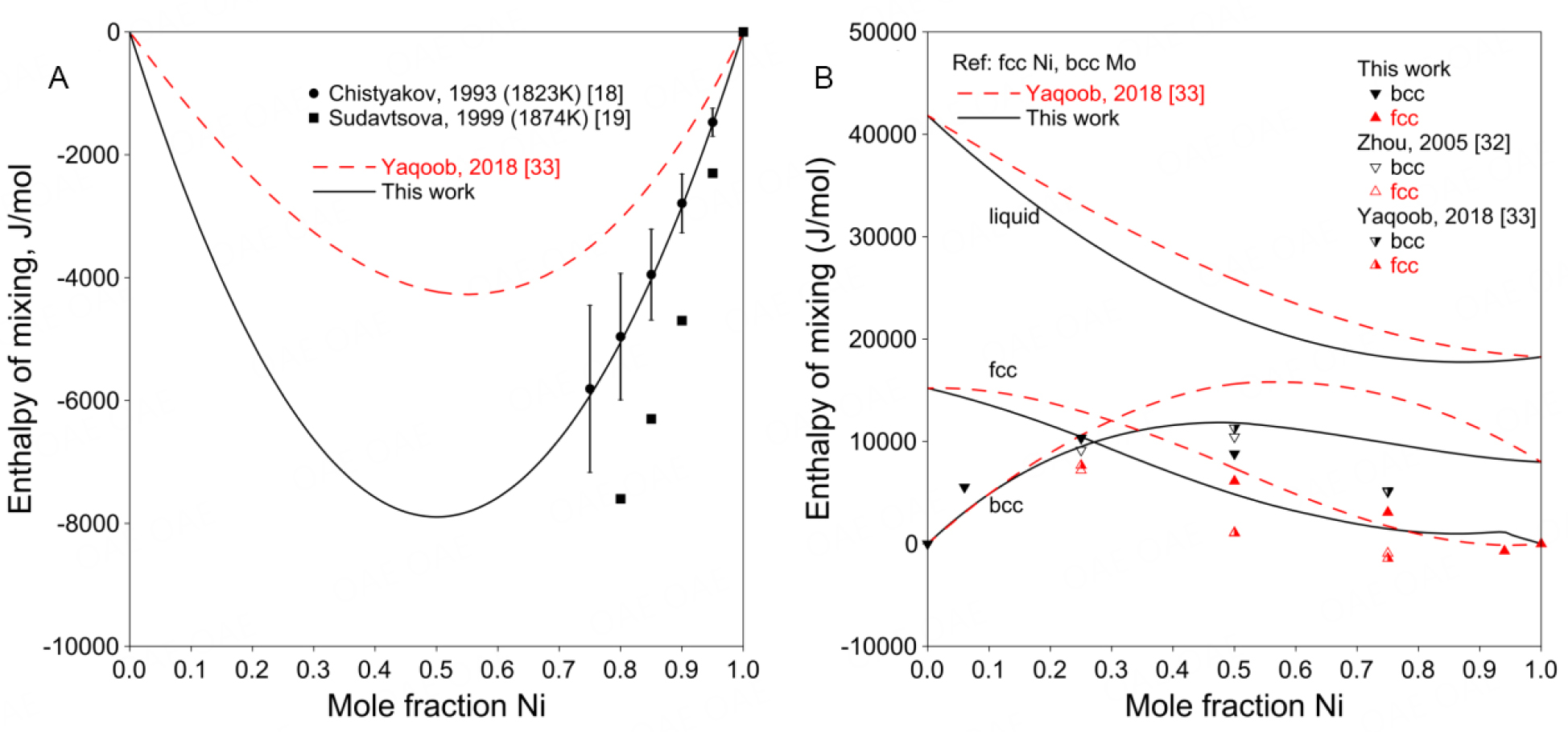Figure 3. (A) Assessed enthalpy of mixing for liquid at 1873 K compared with experimental data. (B) Assessed enthalpy of mixing for solution phases at 298 K compared with SQS results for Ni-Mo system.

Figure 3B shows the assessed enthalpies of mixing for solution phases at 298 K compared with the SQS data[32,33]. As noted above, the SQS calculations were performed only for stable structures in the present work, since possible unstable structures, such as fcc Mo or bcc Ni, may not be maintained during the full relaxation. Therefore, the SQS enthalpies of mixing from Zhou et al. and Yaqoob et al. were not used in the assessment[32,33]. Figure 3B shows a positive enthalpy for the bcc phase, indicating instability at low temperatures, which is in accordance with the phase diagram shown in Figure 2, where there is less than 3.0 at.% Ni soluble in bcc Mo at low temperatures.

Figure 4A shows the assessed formation enthalpies of Ni$$_4$$Mo and Ni$$_3$$Mo compared with the DFT data and previous assessments. Compared to earlier thermodynamic assessments[47,48], the assessed enthalpies of formation in the present work are more reliable with the help of DFT calculation. There are experimental data in Figure 4B showing that the $$C_P$$ of fcc does not follow the Kopp-Neumann rule (red dashed line for fcc), so an extra term CTln(T) was used in order to fit the experimental data. The data measured by Brooks and Meschter deviate largely from the assessment but agree well with the calculated $$C_P$$ at 78 at.% Ni (dotted line). There was probably a deviation in the composition of the sample in the experiments by Brooks and Meschter.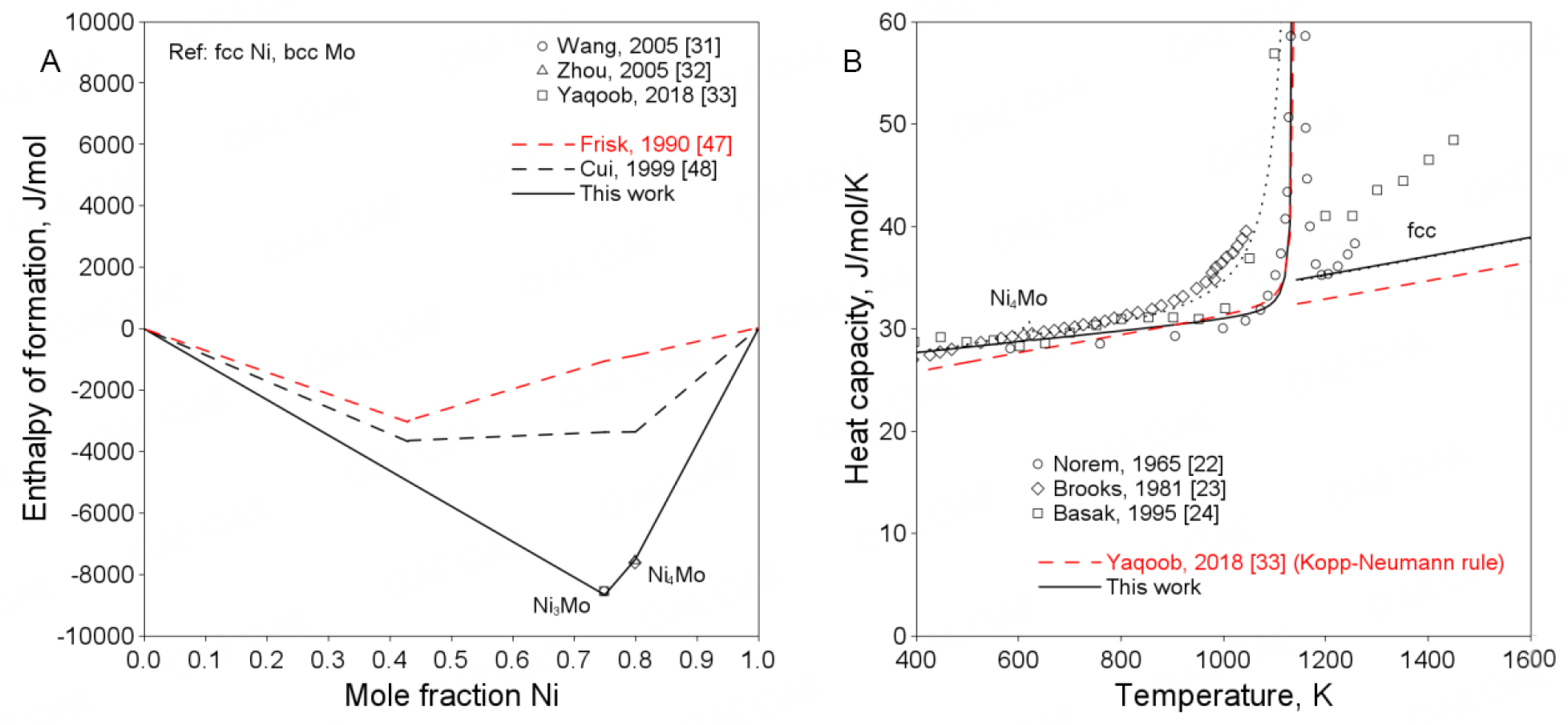Figure 4. (A) Assessed enthalpy of formation at 298 K compared with DFT data. (B) Assessed heat capacities of Ni$$_4$$Mo and fcc at 80 at.% Ni compared with experimental data (dotted line represents the calculated $$C_P$$ at 78 at.% Ni).

### Mo-Re system

Knapton conducted a careful study of this system by means of melting-point measurements, XRD and metallographic methods, using both powder-metallurgical and arc-melting samples. Knapton did not clarify which preparation method was used for each piece of data clearly. However, he showed that between 1673 and 1873 K, only the $$\sigma$$ phase was stable at $$\sim$$68 at.% Re, while in the immediate vicinity of 70 at.% Re, the $$\sigma$$ phase decomposed. This means that the single $$\sigma$$ phase region can reach as far as 68 at.% Re between 1673 and 1873 K. All other available experimental data[3,36,38-40] also manifest a wide single $$\sigma$$ phase region over a wide temperature range in the binary Mo-Re system. In addition, the previously assessed homogeneity range of the $$\sigma$$ phase at low temperatures was so narrow that the extrapolation to the ternary system cannot agree with the experimental data, which will be discussed in the following ternary assessment. Any attempt to reproduce a U-shape $$\sigma$$ phase boundary led to a very poor fit of site occupancy. Considering the wide single $$\sigma$$ phase region over a wide temperature range and the fact of difficulty reaching equilibrium at lower temperatures, the estimated eutectoid temperature may need further confirmation.

As mentioned in the literature review, when extrapolating the Mo-Re system with the parameters of Mathieu et al. to the ternary system, the bcc phase of the three-phase equilibria (i.e., bcc + $$\delta$$ + bct and bcc + bct + $$\sigma$$) has a relatively high Mo content comparing to the experimental data[3,53]. It was found that the Gibbs energy of the binary bcc phase assessed by Mathieu et al. was too positive by giving the sub-regular solution parameter (i.e., $$^1L^{bcc}_{Mo, Re}$$) in order to reduce the binary bcc+$$\sigma$$ two-phase region. Thus, in the present work, a regular solution model was adopted to solve the problem.

Figure 5 shows the present calculated phase diagram of the Mo-Re system and the assessment by Mathieu et al. together with the experimental data[3,36-40,53]. A larger $$\sigma$$ phase region is realized but fails at the Re-rich side as the site occupancy discussed below exerts a constraint. Furthermore, the bcc single phase at 2673 K and $$\sim$$40 at.% Re was not fitted by Mathieu et al. but is well considered in the present work.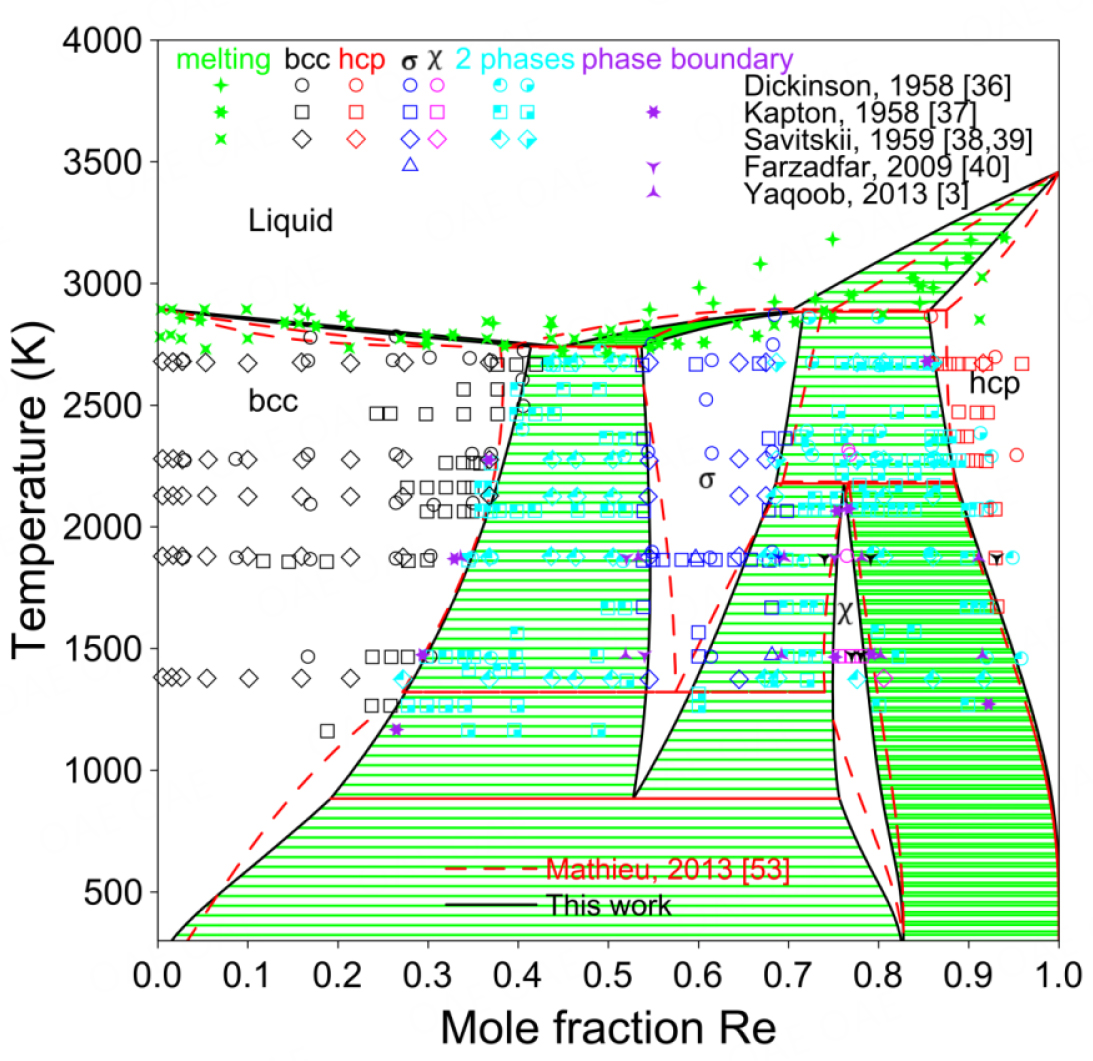Figure 5. Assessed Mo-Re phase diagram compared with experimental data and assessment by Mathieu et al..

Figure 6 shows the calculated enthalpies of mixing at 298 K together with the SQS data from Mathieu et al..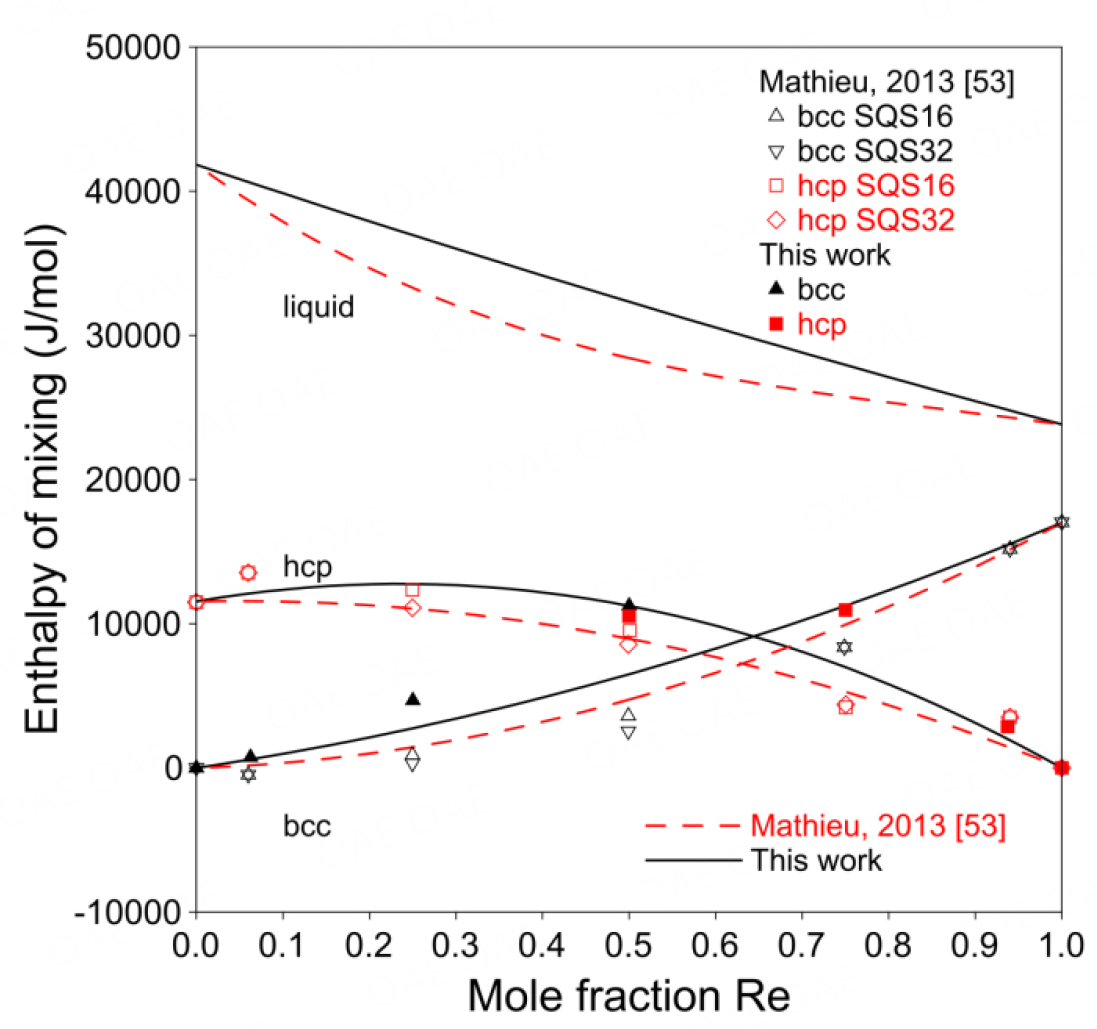Figure 6. Assessed enthalpy of mixing of Mo-Re bcc and hcp phases compared with SQS data.

The present model can well predict the site fractions, as shown in Figure 7. Mathieu et al. did not use excess energy terms for the $$\sigma$$ and $$x$$ phases because they assumed that all the interactions characterizing the phase were taken into account by the DFT calculations. For the $$\sigma$$ phase, the VASP data can predict the site fractions perfectly without any interaction parameter. However, it is necessary to introduce interaction parameters for the $$x$$ phase to fit the site fractions of the 2A and 8C sites.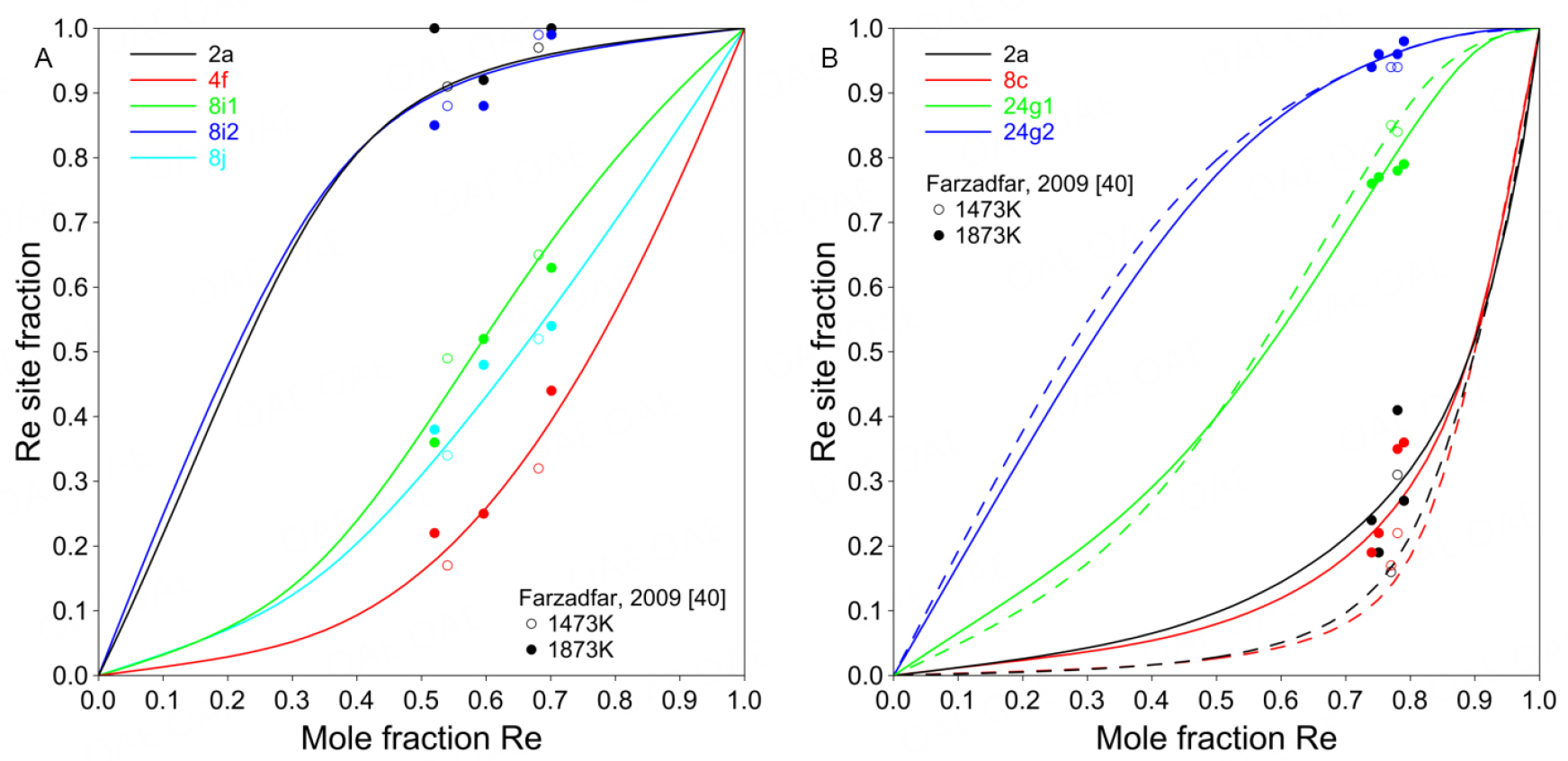Figure 7. Calculated site fractions of Re for (A) Mo-Re $$\sigma$$ and (B) $$x$$ at 1673 K. In (B), the solid lines represent the case considering the interaction parameter L, while dashed lines represent the case without L.

### Ni-Mo-Re system

#### Isothermal sections

Figure 8 compares the assessed isothermal sections at 1425, 1473 and 1873 K with the experimental data. Since the Ni-Mo-Re system was assessed mainly based on the experimental data by Yaqoob et al., it is impossible to reproduce the phase equilibria concerning the $$\sigma$$ phase measured by Borisov et al. at 1425 K[3,42]. For other phase equilibria, there is no conflict between the results reported by Borisov et al. and Yaqoob et al.[3,42]. The calculated isothermal sections present a good agreement with the experimental data, especially the extended $$\sigma$$ single-phase region in the ternary system. The two composition sets for the $$\sigma$$ phase are well reproduced.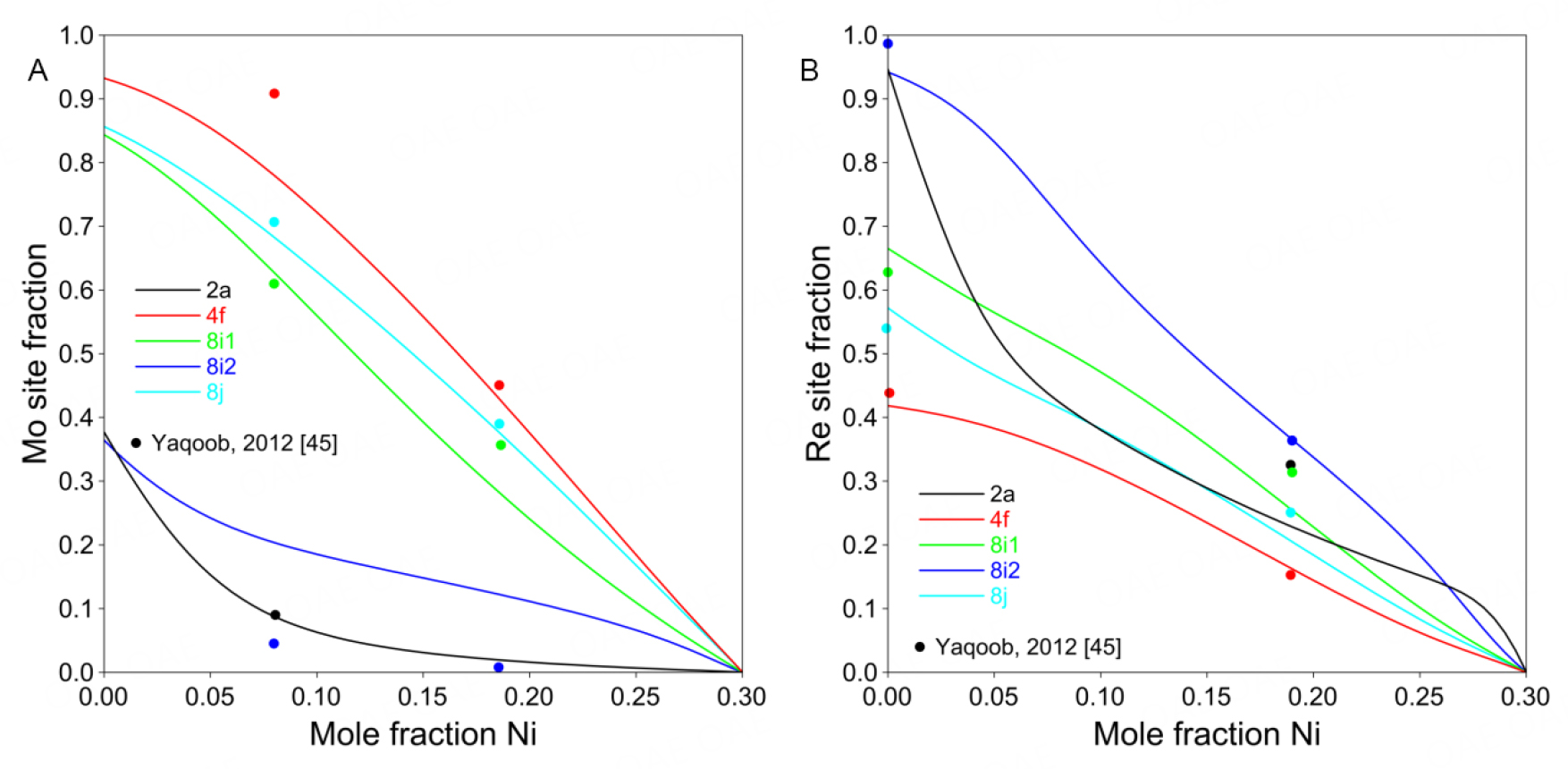Figure 8. Calculated site fractions for Ni-Mo-Re $$\sigma$$ phase at 1873 K for (A) Mo along Mo$$_{0.7}$$Re$$_{0.3}$$-Ni$$_{0.3}$$Re$$_{0.7}$$ and (B) Re along Mo$$_{0.3}$$Re$$_{0.7}$$-Ni$$_{0.3}$$Mo$$_{0.7}$$.

By applying the 5SL model, the site fractions and the ordering behavior of the ternary $$\sigma$$ phase can also be examined as a function of composition and temperature. In Figure 9, the calculated site fractions are in reasonable agreement with the experimental data at 1873 K.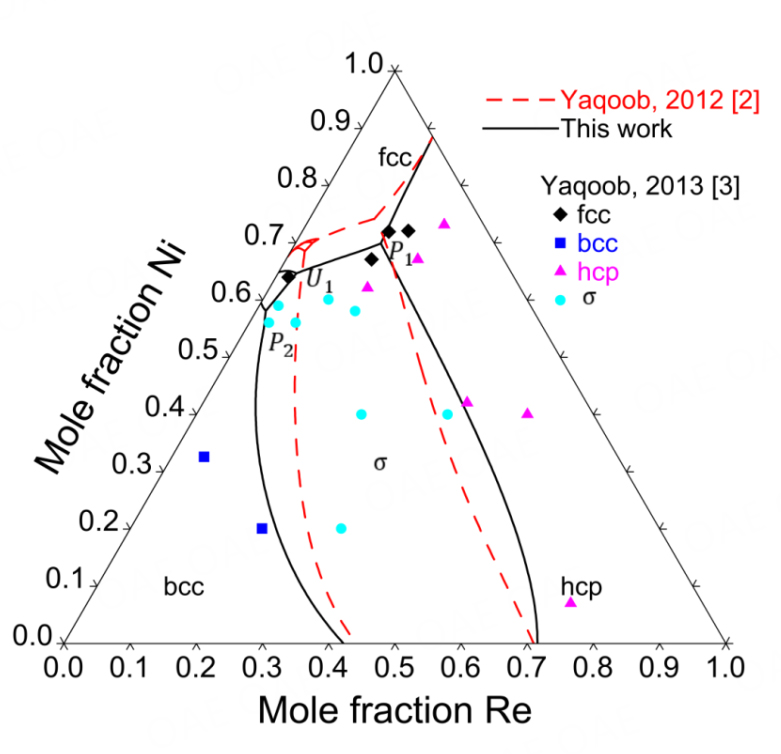Figure 9. Assessed primary crystallization surface of Ni-Mo-Re ternary system compared with experimental data.

#### Primary crystallization surface

The primary crystallization surface was assessed using the experimental data from Yaqoob et al. and presented in Figure 10, where there are five primary crystallization surfaces, namely, fcc, bcc, hcp, $$\delta$$ and $$\sigma$$. It is diﬃcult to perfectly fit the primary surfaces of the hcp and fcc phases since their thermodynamic parameters were primarily determined according to the isothermal sections at 1473 and 1873 K.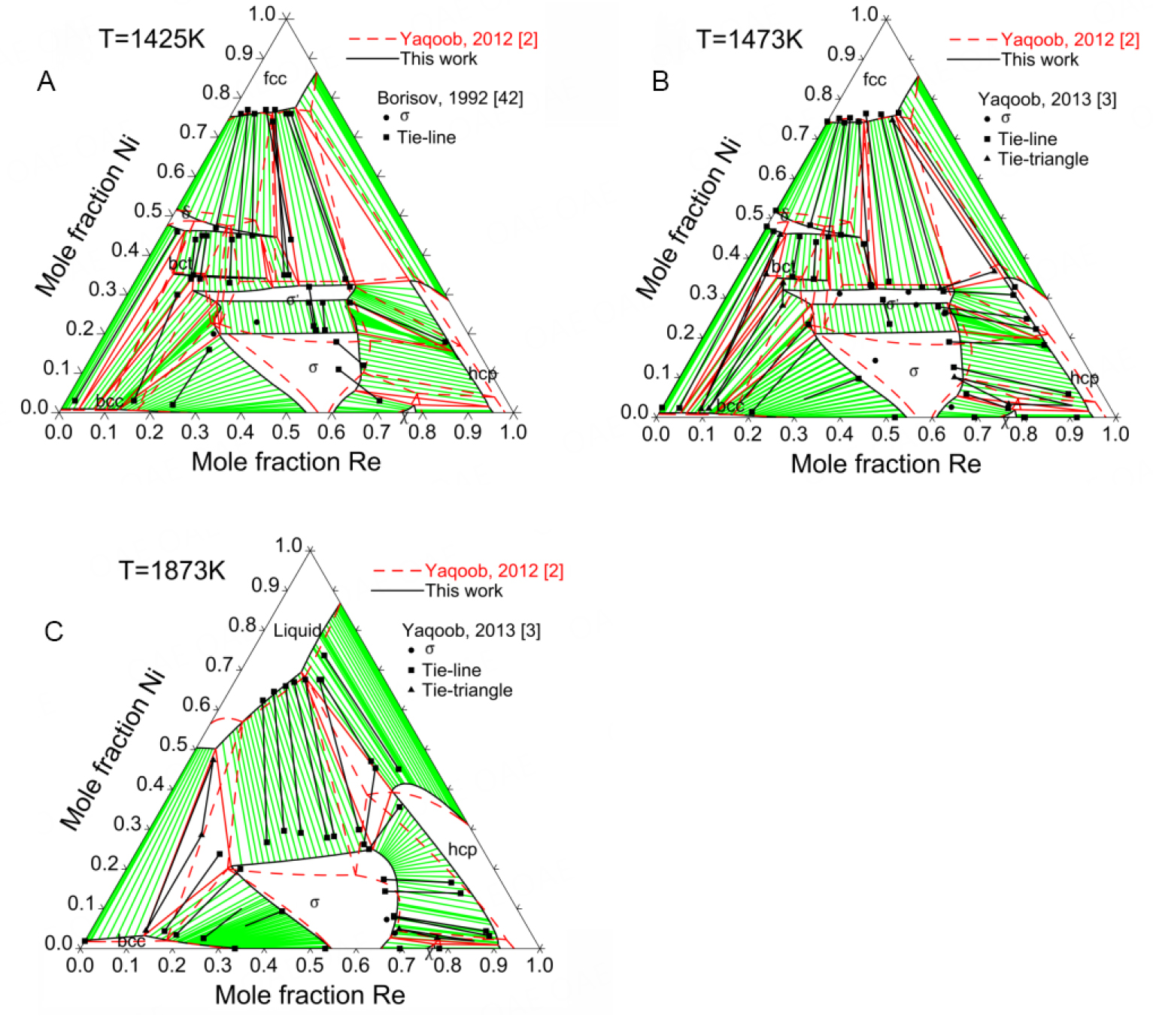Figure 10. Calculated isothermal sections of Ni-Mo-Re ternary system at (A) 1425, (B) 1473 and (C) 1873 together with experimental data.

## CONCLUSIONS

The present work adopts the CALPHAD method to analyze a large amount of experimental and DFT data concerning the thermodynamics and phase diagrams of the Ni-Mo-Re alloy system. It demonstrates that the CALPHAD method can act as a practical appliance in the toolbox of materials informatics to assess various data by consistent modeling and then produce more useful data by computational predictions.

The Ni-Mo and Mo-Re binary systems were reassessed to obtain a better agreement with the experimental data. The large homogeneity range of the $$\sigma$$ phase in the Mo-Re system was examined and considered. For the Ni-Mo-Re ternary system, the reliability of the present model parameters was confirmed by the comprehensive comparison between the assessed and experimental data, i.e., three isothermal sections, site occupancy and primary crystallization surface. In particular, the 5SL modeling of the $$\sigma$$ phase enables a satisfactory description of the co-existence of the $$\sigma$$ phase and its modulated variant $$\sigma$$' structure.

### Appendix I

By using the 5SL model for the sigma phase, positive values of the binary parameters $$^0L^\sigma_{*:*:*:Ni, Mo:*}$$ and $$^0L^\sigma_{*:*:*:Ni, Re:*}$$ ncrease the enthalpies between the end-members NiMoMoMoMo and MoMoMoNiMo for Ni-Mo, NiReReReRe and ReReReNiRe for Ni-Re, respectively. These parameters lead to two composition sets at the low Ni region in the Ni-Mo and Ni-Re binary systems (see the following two figures: left for Ni-Mo, right for Ni-Re).

However, these end-members in the binary systems (such as NiMoMoMoMo and MoMoMoNiMo, see figures below) do not exist when using 3SL, so no interaction parameters can be used to adjust the MG. In conclusion, 3SL cannot reproduce the experimentally determined MG.

### Appendix II

Thermodynamic parameters of the Ni-Mo-Re system assessed in the present work

Liquid

$$^0L^{Liquid}_{Ni, Mo}$$=-31580+9.1T

$$^0L^{Liquid}_{Mo, Re}$$=-2000

$$^0L^{Liquid}_{Ni, Mo, Re}$$=0

$$^1L^{Liquid}_{Ni, Mo, Re}$$=0

$$^2L^{Liquid}_{Ni, Mo, Re}$$=-30000

fcc

$$^0L^{fcc}_{Ni, Mo}$$=-17930+119.69T-15.1T$$\ln$$T

$$^1L^{fcc}_{Ni, Mo}$$=12700

$$\beta^{fcc}_{Ni, Mo}$$=-3.5

$$^1T_{C Ni, Mo}^{fcc}$$=-5500

$$^0L^{fcc}_{Ni, Mo, Re}$$=87840-85T

$$^1L^{fcc}_{Ni, Mo, Re}$$=97840-80T

$$^2L^{fcc}_{Ni, Mo, Re}$$=0

bcc

$$^0L^{bcc}_{Ni, Mo}$$=27191

$$^1L^{bcc}_{Ni, Mo}$$=18792

$$^0L^{bcc}_{Mo, Re}$$=-8000+3.5T

$$^0L^{bcc}_{Ni, Mo, Re}$$=40380-20T

$$^1L^{bcc}_{Ni, Mo, Re}$$=0

$$^2L^{bcc}_{Ni, Mo, Re}$$=50000

hcp

$$^0L^{hcp}_{Mo, Re}$$=21850-5.2T

$$^0L^{hcp}_{Ni, Mo, Re}$$=243650-50T

$$^1L^{hcp}_{Ni, Mo, Re}$$=160950-150T

$$^2L^{hcp}_{Ni, Mo, Re}$$=-60000

$$\delta$$

$$^0G^\delta_{Ni:Mo:Re}$$=24$$^0G^{fcc}_{Ni}+32^0G^{bcc}_{Mo}-238250+1397T-185T \ln (T)$$

$$^0G^\delta_{Ni:Ni:Mo}$$=44$$^0G^{fcc}_{Ni}+12^0G^{bcc}_{Mo}-74620+2340T-292T \ln (T)$$

$$^1G^\delta_{Ni:Ni:Mo:Mo}$$=-294600+450T

$$^0G^\delta_{Ni:Re:Mo}$$=24$$^0G^{fcc}_{Ni}+20^0G^{bcc}_{Mo}-12^0G^{hcp}_{Re}-329112$$

$$^0G^\delta_{Ni:Ni:Re:Mo}$$=302700

$$\mathbf{Ni_3Mo}$$

$$^0G^{Ni_3Mo}_{Ni:Ni}=4^0G^{fcc}_{Ni}+11360+15T$$

$$^0G^{Ni_3Mo}_{Ni:Mo}=3^0G^{fcc}_{Ni}+^0G^{bcc}_{Mo}-40160+23.2T$$

$$^0G^{Ni_3Mo}_{Ni:Ni}=3^0G^{bcc}_{Mo}+^0G^{fcc}_{Ni}+68240$$

$$^0G^{Ni_3Mo}_{Mo:Mo}=4^0G^{bcc}_{Mo}+170600$$

$$\mathbf{Ni_4Mo}$$

$$G^{Ni_4Mo}_{m}=-76820+734T-128T\ln(T)-0.013T^2$$

$$\sigma$$

$$\triangle S^{\sigma -SER}_{Ni}$$=2.4

$$\triangle S^{\sigma -SER}_{Mo}$$=-1.5

$$\triangle S^{\sigma -SER}_{Re}$$=-1.2

$$^0L^\sigma_{*:*:*:Ni, Mo:*}$$=20700

$$^0L^\sigma_{*:*:*:Ni, Re:*}$$=20700

$$^0L^\sigma_{*:*:*:Ni, Mo, Re:*}$$=-1050000

$\chi  \triangle S^{\chi -SER}_{Ni} $$=1.3$$ \triangle S^{\chi -SER}_{Mo} $$=-2.42$$ \triangle S^{\chi -SER}_{Re} $$=0.85$$ ^0L^\chi_{Mo, Re:*:*:*} $$=-30T$$ ^0L^\chi_{*:Mo, Re:*:*} $$=-100T$$ ^0L^\chi_{*:*:Mo, Re:*} $$=-100T bct$$ ^0G^{bct}_{Mo:Ni:Ni}=9^0G^{bcc}_{Mo}+5^0G^{fcc}_{Ni}-22110-7T  ^0G^{bct}_{Re:Ni:Ni}=9^0G^{hcp}_{Re}+5^0G^{fcc}_{Ni}-7812+20T  ^0G^{bct}_{Mo:Re:Ni}=9^0G^{bcc}_{Mo}+4^0G^{hcp}_{Re}+^0G^{fcc}_{Ni}+69748  ^0G^{bct}_{Re:Re:Ni}=13^0G^{hcp}_{Re}+^0G^{fcc}_{Ni}+153020  L^{bct}_{Mo, Re:*:Ni}=-120000$$## DECLARATIONS ### Authors' contributions Writing and editing: Lei XH, Lu XG First-principles calculations: Liu W Data analysis and discussion: Lei XH, Liu W, Luo F, Lu XG ### Availability of data and materials Supplementary materials are available from the Journal of Materials Informatics or from the authors. ### Financial support and sponsorship The present work is supported by the National Key R & D Program of China (Grant No. 2017YFB0701502). ### Conflicts of interest The authors declared that there are no conﬂicts of interest. ### Ethical approval and consent to participate Not applicable. ### Consent for publication Not applicable. ### Copyright © The Author(s) 2022. ### Supplementary Materials ## References • 1. Reed RC. The superalloys fundamental and applications New York: Cambridge University Press; 2008. DOI • 2. Yaqoob K. Thesis. Paris-Est Créteil University, 2012. Available from: https://www.theses.fr/2012PEST1172.pdf[Last accessed on 29 Jul 2022]. • 3. Yaqoob K, Joubert J. Experimental investigation of the Mo-Ni-Re system. J Alloys Compd 2013;559:101-11. DOI • 4. J. -C. Crivello. Unpublished work. • 5. Yaqoob K, Guénée L, Černý R, Joubert J. A modulated structure derived from the$\sigma$phase in the Mo-Ni-Re system. Intermetallics 2013;37:42-5. DOI • 6. Yaqoob K, Joubert J. Experimental determination and thermodynamic modeling of the Ni-Re binary system. J Solid State Chem 2012;196:320-5. DOIPubMed • 7. Grube G, Vosskühler H. Elektrische Leitfähigkeit und Zustandsdiagramm bei binären Legierungen. 8. Mitteilung. Das System Lithium-Zink. Z Anorg Allg Chem 1933;215:211-24. DOI • 8. Bloom DS, Grant NJ. An investigation of the systems formed by chromium, molybdenum, and nickel. JOM 1954;6:261-8. DOIPubMed • 9. P.V. Guthrie, E.E. Stansbury, X-ray and metallographic study of the nickel-rich alloys of the nickel-molybdenum system. Ⅱ, Oak Ridge National Laboratory, Oak Ridge, Tennessee, 1961, p. 57. DOI • 10. Casselton R, Hume-rothery W. The equilibrium diagram of the system molybdenum-nickel. J Less Comm Metals 1964;7:212-21. DOI • 11. A. Wicker, C. Allibert, J. Driole, E. Bonnier, Etude d' équilibres de phases dans les systèmes Ni-Nb-Mo, Ni-Nb et Ni-Mo, Comptes Rendus l'Acad. Sci. Sér. C 271 (1970) 273-275. DOI • 12. Heijwegen CP, Rieck GD. Determination of the phase diagram of the molybdenum-nickel system using diffusion couples and equilibrated alloys. Inter J Mater Res 1973;64:450-3. DOI • 13. W. Gust, T. Nguyen-Tat, B. Predel, untersuchungen zur dikontinuierlichen ausscheidung in einer Ni-Mo-Legierung mit 17.5 at. % Mo, Z. Metallkd. 70 (1979) 241-246. DOI • 14. Kang SL, Song Y, Kaysser WA, Hofmann H. Determination of Mo solidus in the Mo-Ni system by electrolytic phase separation method. Inter J Mater Res 1984;75:86-91. DOI • 15. Kobayashi S, Sumi T, Koyama T, Miyazaki T. Determination of coherent phase boundaries in Ni-V and Ni-Mo alloys by utilizing macroscopic composition gradient. J Japan Inst Metals 1996;60:22-8. DOI • 16. Zhu L, Wei C, Jiang L, Jin Z, Zhao J. Experimental determination of the phase diagrams of the Co-Ni-X (X = W, Mo, Nb, Ta) ternary systems using diffusion multiples. Intermetallics 2018;93:20-9. DOI • 17. Shi J, Guo C, Li C, Du Z. Experimental investigation of intermetallics and phase equilibria in the Hf-Mo-Ni system at 1100 ℃ and 950 ℃. Metall Mater Trans A 2021;52:1059-76. DOI • 18. ChistyakovLS, Grigorovich KV, Stomakhin AY. Enthalpy of formation of liquid Ni-Mo alloys, Izv. Vyss. Uchebn. Zaved. Chern. Metall 1993:82-83. DOI • 19. Sudavtsova VS. Thermodynamic properties of melts of Ni-Cr(Mo, W) binary systems, Russ. Metall 1999:118-120. DOI • 20. Spencer P, Putland F. A calorimetric study of intermediate phases in iron +, cobalt +, and nickel + molybdenum. J Chem Thermodyn 1975;7:531-6. DOI • 21. Kubaschewski O, Hoster T. Bildungs- und Umwandlungs-Enthalpien in binären und ternären Systemen der Metalle Eisen, Kobalt, Nickel und Molybdän. International J Mater Res 1983;74:607-9. DOI • 22. Norem, WE, Ph. D. Thesis. University of Tennessee, Knoxville, 1965. • 23. Brooks CR, Meschter PJ. A Combined Thermodynamic Study of Nickel-Base Alloys, Department of Energy, Knoxville, Tennessee, 1981, p. 42. DOI • 24. Basak D. Application of Pulse Calorimetry to Metal Systems, (n. d. ) 248. DOI • 25. Katayama I, Shimatani H, Kozuka Z. Thermodynamic study of solid Cu-Ni and Ni-Mo alloys by E.M.F. Measurements using the solid electrolyte. J Japan Inst Metals 1973;37:509-15. DOI • 26. Meshkov LL, Guzei LS, Sokolovskaya EM. Sokolovskaia, thermodynamics of nickel-molybdenum alloys. Russ J Phys Chem 1975;49: 1128-9. Available from: https://www.osti.gov/biblio/4035422[Last accessed on 29 Jul 2022]. • 27. Tsai HL. Thesis, University of Tennessee, 1983. • 28. Pejryd L. Experimental study of phase equilibria and thermodynamic stabilitie in the system Ni-Mo-TiO2-O, Department of Inorganic Chemistry, University of Umeå, Umeå, Sweden, 1985. • 29. Pejryd L. Phase relations and stabilities in the metal-rich part of the Ni-Mo-O system in the temperature range 1200-1400 K. Scand J Metall 1985;14:268-272. DOI • 30. Koyama K, Hashimoto Y, Suzuki K, Kameyama S. Determination of the standard gibbs free energy of formation of NiMo$_2$B$_2$and the activity of the Ni-Mo binary system by EMF measurement. J Japan Inst Metals 1989;53:183-8. DOI • 31. Wang Y, Woodward C, Zhou S, Liu Z, Chen L. Structural stability of Ni-Mo compounds from first-principles calculations. Scripta Materialia 2005;52:17-20. DOI • 32. Zhou S, Wang Y, Jiang C, Zhu J, Chen L, Liu Z. First-principles calculations and thermodynamic modeling of the Ni-Mo system. Mater Sci Eng 2005;397:288-96. DOI • 33. Yaqoob K, Crivello J, Joubert J. Thermodynamic modeling of the Mo-Ni system. Calphad 2018;62:215-22. DOI • 34. Khan F, Asgar M, Nordblad P. Magnetization and magnetocrystalline anisotropy of Ni-Mo single crystal alloys. J Magn Magn Mater 1997;174:121-6. DOI • 35. Ghosh S, Das N, Mookerjee A. Magnetic properties of Ni-Mo single-crystal alloys; theory and experiment. J Phys : Condens Matter 1998;10:11773-80. DOI • 36. J.M. Dickinson, L.S. Richardson, Trans. ASM 51 (1958) 1055-1071. DOI • 37. A.G. Knapton, J. Inst. Met. 87 (1958-1959) 62-64. • 38. E.M. Savitskii, M.A. Tylkina, K.B. Povarova, Zh. Neorg. Khim. 1959;4: 424-34. • 39. E.M. Savitskii, M.A. Tylkina, K.B. Povarova, Russ. J. Inorg. Chem. 4 (1959) 190-195. • 40. Farzadfar S, Levesque M, Phejar M, Joubert J. Thermodynamic assessment of the molybdenum - rhenium system. Calphad 2009;33:502-10. DOI • 41. Kodentsov AA, Dunae SF, Slyusarenko EM, Sokolovskaya EM, Priimak AN. Phase equilibria in the rhenium-molybdenum-nickel system. Vestnik Moskovskogo Universiteta Ser. 2 Khimiya, 1987;28: 153-8. DOI • 42. Borisov VA, Yaschenko AI, Slyusarenko EM, Dunaev SF. Moscow Univ. Chem. Bull. 1992;47: 76-9. • 43. Slyusarenko E, Borisov V, Sofin M, Kerimov E, Chastukhin A. Determination of phase equilibria in the system Ni-V-Cr-Mo-Re at 1425 K using the graph method. J Alloys Compd 1999;284:171-89. DOI • 44. Feng Y. Determination of isothermal sections of the Co-Nb-Ni and Ni-Mo-Re ternary systems. Rare Metals 2008;27:83-8. DOI • 45. Yaqoob K, Crivello JC, Joubert JM. Comparison of the site occupancies determined by combined Rietveld refinement and density functional theory calculations: example of the ternary Mo-Ni-Re$\sigma\$ phase. Inorg Chem 2012;51:3071-8.

DOI
• 46. Chekhovskoi VY, Peletskii VE. Thermophysical properties of 75Ni-15Mo-10Re alloy. High Temperature 2003;41:221-8.

DOI
• 47. Frisk K. A thermodynamic evaluation of the Mo-Ni system. Calphad 1990;14:311-20.

DOI
• 48. Cui Y, Jin Z, Lu X. Experimental study and thermodynamic assessment of the Ni-Mo-Ta ternary system. Metall Mater Trans A 1999;30:2735-44.

DOI
• 49. Morishita M, Koyama K, Yagi S, Zhang G. Calculated phase diagram of the Ni-Mo-B ternary system. J Alloys Compd 2001;314:212-8.

DOI
• 50. Mao P, Han K, Xin Y. Thermodynamic assessment of the Mo-Re binary system. J Alloys Compd 2008;464:190-6.

DOI
• 51. Mao P, Han K, Xin Y. Corrigendum to "thermodynamic assessment of the Mo-Re binary system". J Alloys Compd 2009;482:557-8.

DOI
• 52. Yang Y, Zhang C, Chen S, Morgan D, Chang YA. First-principles calculation aided thermodynamic modeling of the Mo-Re system. Intermetallics 2010;18:574-81.

DOI
• 53. Mathieu R, Dupin N, Crivello J, et al. CALPHAD description of the Mo-Re system focused on the sigma phase modeling. Calphad 2013;43:18-31.

DOI
• 54. Nash A, Nash P. Ni-Re (Nickel-Rhenium) system. Bull Alloy Phase Diagrams 1985;6:348-50.

DOI
• 55. Boettinger WJ, Newbury DE, Ritchie NWM, et al. Solidification of Ni-Re peritectic alloys. Metall Mat Trans A 2019;50:772-88.

PubMed PMC
• 56. Kresse G, Furthmüller J. Efficient iterative schemes for ab initio total-energy calculations using a plane-wave basis set. Phys Rev B Condens Matter 1996;54:11169-86.

DOIPubMed
• 57. Dreizler RM, Gross EKU. Density functional theory. Springer Berlin Heidelberg: Berlin, Heidelberg, 1990; Chapter 4, pp 43-74.

DOI
• 58. Kresse G, Joubert D. From ultrasoft pseudopotentials to the projector augmented-wave method. Phys Rev B 1999;59:1758-75.

DOI
• 59. Perdew JP, Wang Y. Accurate and simple analytic representation of the electron-gas correlation energy. Phys Rev B Condens Matter 1992;45:13244-9.

DOIPubMed
• 60. Monkhorst HJ, Pack JD. Special points for Brillouin-zone integrations. Phys Rev B 1976;13:5188-92.

DOI
• 61. Blöchl PE. Projector augmented-wave method. Phys Rev B Condens Matter 1994;50:17953-79.

DOI
• 62. Zunger A, Wei S, Ferreira LG, Bernard JE. Special quasirandom structures. Phys Rev Lett 1990;65:353-6.

DOIPubMed
• 63. van de Walle A, Tiwary P, de Jong M, et al. Efficient stochastic generation of special quasirandom structures. Calphad 2013;42:13-8.

DOI
• 64. Shin D, Arróyave R, Liu Z, Van de Walle A. Thermodynamic properties of binary hcp solution phases from special quasirandom structures. Phys Rev B 2006;74:024204.

DOI
• 65. Crivello J, Souques R, Breidi A, Bourgeois N, Joubert J. ZenGen, a tool to generate ordered configurations for systematic first-principles calculations: the Cr-Mo-Ni-Re system as a case study. Calphad 2015;51:233-40.

DOI
• 66. Sundman B, Shi P. SGTE pure element database, version 5.0, Thermo-Calc Software, Stockholm.

• 67. Zheng W, Lu X, He Y, Cui Y, Li L. Thermodynamic assessment of the Fe-Mn-Si system and atomic mobility of its fcc phase. J Alloys Compd 2015;632:661-75.

DOI
• 68. Redlich O, Kister AT. Thermodynamics of nonelectrolyte solutions - x-y-t relations in a binary system. Ind Eng Chem 1948;40:341-5.

DOI

OAE Style

Lei XH, Liu W, Luo F, Lu XG. A thermodynamic database of the Ni-Mo-Re system. J Mater Inf 2022;2:11. http://dx.doi.org/10.20517/jmi.2022.15

AMA Style

Lei XH, Liu W, Luo F, Lu XG. A thermodynamic database of the Ni-Mo-Re system. Journal of Materials Informatics. 2022; 2(3):11. http://dx.doi.org/10.20517/jmi.2022.15

Chicago/Turabian Style

Lei, Xiong-Hui, Wei Liu, Fenghua Luo, Xiao-Gang Lu. 2022. "A thermodynamic database of the Ni-Mo-Re system" Journal of Materials Informatics. 2, no.3: 11. http://dx.doi.org/10.20517/jmi.2022.15

ACS Style

Lei, X.H.; Liu W.; Luo F.; Lu X.G. A thermodynamic database of the Ni-Mo-Re system. J. Mater. Inf. 20222, 11. http://dx.doi.org/10.20517/jmi.2022.15

Views
293
251
Citations0
0

1

• Cited:0